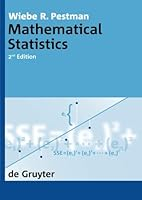# Mathematical Statistics, 2nd Edition1 Reviews
2009-04-20
597 pages

## Book Description

This textbook provides a broad and solid introduction to mathematical statistics, including the classical subjects hypothesis , normal regression , and normal of variance. In addition, non-parametric statistics and vectorial statistics are considered, as well as applications of stochastic in modern statistics, e.g., Kolmogorov-Smirnov , smoothing techniques, robustness and estimation. For students with some elementary mathematical background. With many exercises. Updated, complete Solutions Manual available on request Prerequisites from measure and linear are presented.

Chapter 1. Probability theory
Chapter 2. Statistics and their probability distributions, estimation theory
Chapter 3. Hypothesis tests
Chapter 4. Simple regression analysis
Chapter 5. Normal analysis of variance
Chapter 6. Non-parametric methods
Chapter 7. Stochastic analysis and its applications in statistics
Chapter 8. Vectorial statistics
Appendix A. Lebesgue’s convergence theorems
Appendix B. Product measures
Appendix C. Conditional probabilities
Appendix D. The characteristic function of the Cauchy
Appendix E. Metric spaces, equicontinuity
Appendix F. The Fourier transform and the existence of stoutly tailed distributions

## Book Details

• Title: Mathematical Statistics, 2nd Edition
• Author:
• Length: 597 pages
• Edition: 2
• Language: English
• Publisher:
• Publication Date: 2009-04-20
• ISBN-10: 3110208520
• ISBN-13: 9783110208528